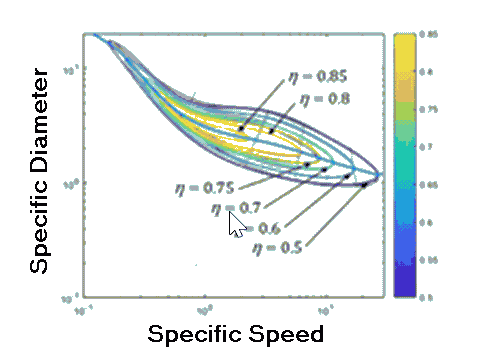Contents - index

Compressor5_CLThe function Compressor5_CL(n_s, d_s) returns isentropic efficiency of a compressor given the specific speed and specific diameter.  The function uses a curve fit to the Balje curves to provide the efficiency of a compressor given the specific speed and specific diameter.  These curves were developed in:

Sondelski, Becky, Mass Optimization of a Supercritical CO2 Brayton Cycle with a Direct Cooled Nuclear Reactor for Space Surface Power, M.S. Thesis, Dept. of Mechanical Engineering, UW-Madison, (2019).

and are based on the Balje curves presented in Balje, O.E., Turbomachinery, New York, Wiley & Sons, (1981).

The calling protocol is:

Compressor5_CL(n_s, d_s)

Inputs:

n_s = specific speed (rad) = omega*sqrt(V_dot)/Dh_s^0.75

d_s = specific diameter (-) = D*Dh_s^0.25/sqrt(V_dot)

eta_max = desired maximum efficiency - use if you want to adjust the maximum possible efficiency, otherwise set to any negative number (Balje curve predicts a maximum possible efficiency of 87.105%)

Output:

Isentropic efficiency

Note that the companion function Compressor6_CL provides the optimal specific diameter at a given value of specific speed in order to stay on the peak of the Balje curve.

Example:

n_s=2

d_s=1.5

eta_max=-999

eta=compressor5_CL(n_s, d_s, eta_max)

{eta = 0.5611}